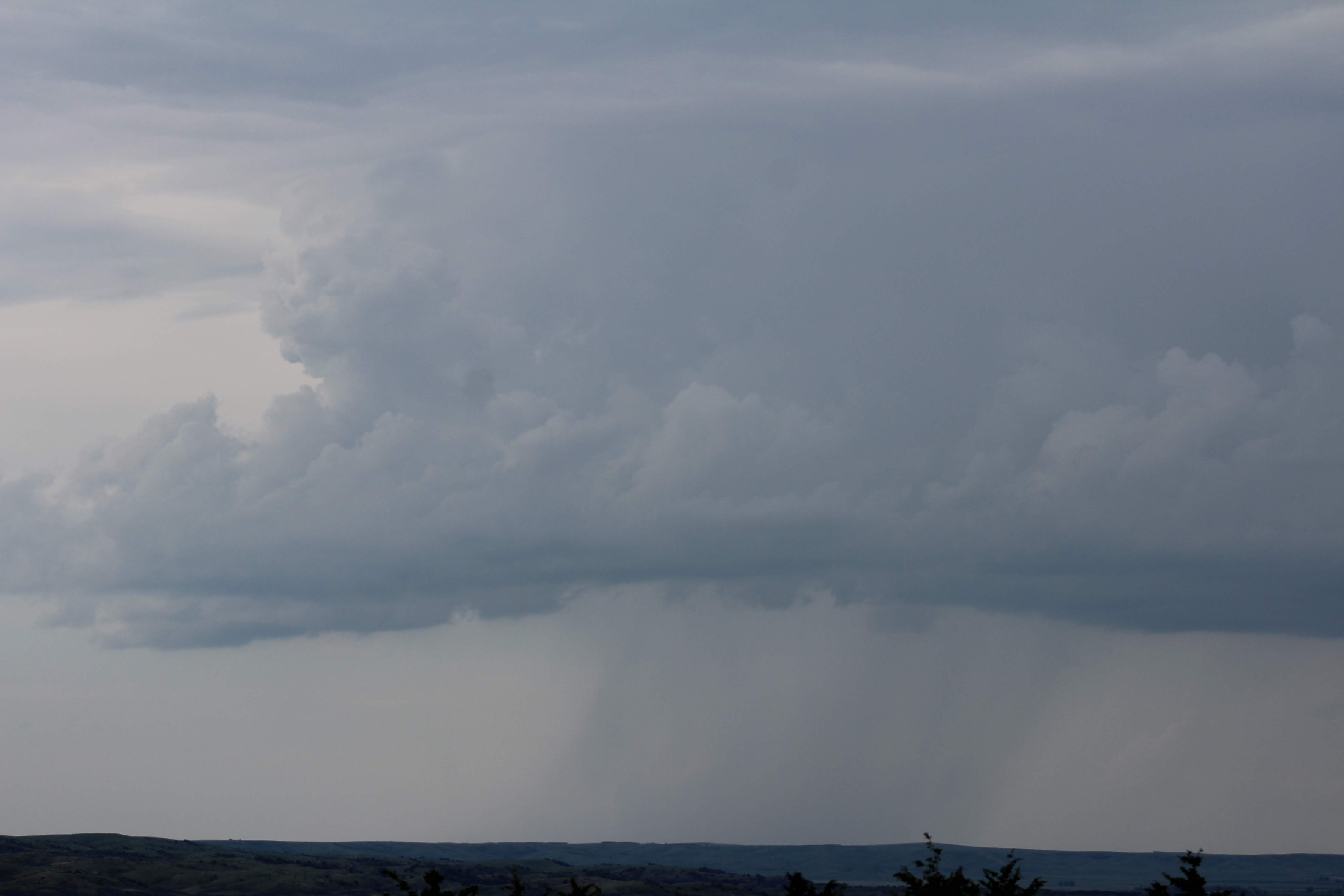What Does Probability of Precipitation Mean?

September 7, 2021 // Article by: Bobby Bianco

We all have heard a meteorologist say there is a "30% chance of rain" on any given day, but what does this mean?

The chance of precipitation or "Probability of Precipitation" (PoP) is a term used by meteorologists to help clients or viewers get an understanding for how likely a specific area is to see rain. Determining the PoP is actually an equation (Forecaster Confidence x Areal Coverage = PoP). For example, in the summer, more often than not there will be a chance for thunderstorms if it is hot and humid. Let's say there is a forecast that has gone out for a 100 square mile area. Thunderstorms are expected to develop in the afternoon and may only impact 30% of the area. We are confident that storms will develop so that might be 80% confidence, but the area they cover is 30%. Since these are both percentages, you calculate 0.80 * 0.30 = 0.24 (then multiply by 100 to get your precentage of 24%). This is a 24% chance of rain in one specific location within the 100 square mile area. This can increase or decrease depending on where the location is. There may be a greater risk farther north, so the confidence and areal coverage may increase depending on where you move. This is also why smaller/more specific forecasts tend to be more "accurate" than a large areal forecast.

Now that we know what goes into the PoPs, does this percentage indicate whether the rain will be heavy or light? The answer to this is actually no. If it's the middle of the summer and you see 30% chance of thunderstorms, if one of these storms moves overhead, your area can pick up a quick 0.50" of rain. However, if there is an pocket of light rain already developed and moving towards the area, the rain chance can be 80% but since it is light, there might only be 0.10" in a couple of hours. An increased PoP usually does not correlate to increased amount of rain since they are different parameters.

With this fully squared away now, let's briefly get into some terminology. Certain PoP values do come with certain terms that can be used. Just for a reference, the terms are illustrated below with their appropriate PoPs.

10 - 25% = Isolated, stray, spotty, can't be ruled out

25 - 60% = Scattered